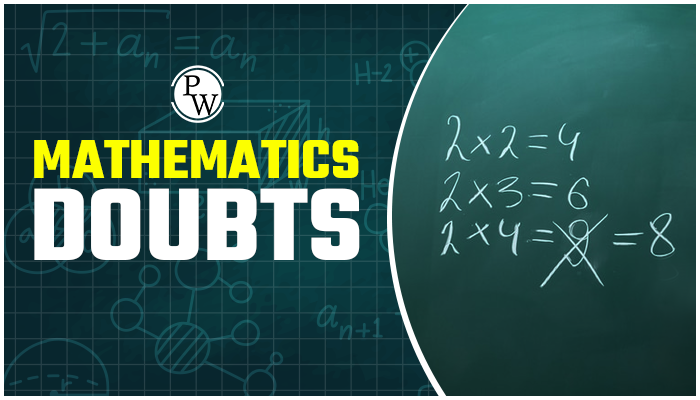# Mathematics Doubts

Solving the questions is best approach for subject like maths.While solving questions in maths one required concept clearity in maths theory and Maths formula.Maths doubts are most common among students and everyone has a unique Maths doubts and method to find the solutions of his her maths doubts.In this page academic team of Physics Wallah collected the most common area in maths on which maximum students face problem and try to elaborate that particular problem we have tried to explain the entire theory of topic on which students face problem.

Physics Wallah team explains theory with solved example to help you to find the solutions of your maths doubts. Do solve NCERT text book with the help of NCERT Solutions for Class 12 Maths.

## How to Handle Maths doubts

When you are in learning phase of mathematics maths doubts are obvious so don’t worry about it and try to solve more and more questions.Addressing maths doubts are very important to get the answer of your maths questions in time will help you to enhanced your learning in subjects like maths.let’s discuss the best approach to develop your concept in maths.

Start with the reading theory of the chapter make sure to make a proper note of the chapter, write down all important theory part with the required derivation and try to make a separate note for Math Formulas .Writing maths formulas in separate note book will help you a lot in the revision and it will help you to retain important formulas in future too which are applicable in all class of maths.It is observed that most of maths doubts arises while solving the numerical of maths , and most of doubts of such types can easily be solved by the solutions of such questionsAcademic team of Physics Wallah uploaded solutions of most of the text book it is highly recommended that students must solve NCERT text book.And solve the questions given in NCERT text book if your face any problem while solving questions of NCERT text book of maths you can take help from NCERT solutions for class 10 maths.NCERT solutions for class 11 maths, NCERT Solutions for class 12 maths. To build a good foundation it is highly recommend solving additional questions from reference book.To help you our academic team of Physics Wallah uploaded a large number of solved and unsolved questions including objective questions with solution and answer key and solutions.

### Mathematics Need Numerical Approach

While solving numerical in maths students face two types of problem because of which generally they did mistake one is unable to understand what is asked in questions which we called as misunderstood the questions and second is forget the formula or concept.To avoid this one must have a good practice in numerical. Read the theory carefully make a good notes for the theory part of the concept try to note down all notes in your note book and if possible keep all class maths notes with you in future classes too.Because maths is subject which is used in every class and class 8 maths is related to class 9 and so on,keeping every class note with you will help you a lot and hep you to clear the maths doubts instantly. Do Follow Maths Symbols.

### Theoretical Math doubts

This is the most important maths doubt and need to be clear very urgently.To get the answer of your maths doubts which are because of theory means you are unable to understand the theory part from the text or the theory mentioned in your class . Don’t worry it’s the most common problem which students face you can resolve such maths doubts in three different ways.

1. Ask in Physics Wallah forum we will help you else search the topic in Physics Wallah you will get the detail theory of this topics.
2. Try to find 10 to 15 solved example and understand the solutions given in these problem , sometime numerical solutions help us to understand the concept which we can’t able to learn from the theory part .
3. Discuss such concepts with your class teacher or friends discussion with friend lead to clear many maths doubts and help you to explore the untouched part of the chapters.

Q1. What is Mathematics and Maths doubt section can help you ?

Ans. Mathematics is the subject that is all around us, in everything we do. It is one of the most important subjects in a student’s life. It is not a subject for reading or just listening, it needs revision for memorizing all concepts It is used in our daily lives, including mobile devices, architecture, art, money, engineering, and even sports.In this section you can ask your Maths question and Physics Wallah experts will answer your doubts in detail.

Q2. Why do we study Maths?

Ans. Maths is essential for knowing the time.

• It helps us in balancing our budgets.
• It helps you in the shopping area, kitchen area, professional area, and other areas in your life.

Q3. How to finish the maths exam on time with all Maths doubt clear?

Ans. Here are some tips –

• Firstly understand the exam pattern if you familiarised with the pattern then you won’t find any difficulty to understand the structure of the paper.
• Prepare a strategy for the exams.
• Use the first 15 minutes, these 15 minutes can be of great help in preparing a strategy for writing the exam correctly.
• Understand the worth of questions.
• Maintain speed and accuracy.

Q4. How important questions and Maths doubt help in a Maths exam?

Ans. Important questions provide you chapter-wise preparation strategies. It will also help you do well in the final exam and prepare for future exams. This will describe your math skills and help you get one step ahead. Practicing important questions covers all concepts and help to achieve good marks.